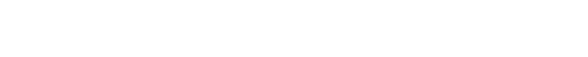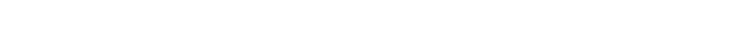# Primary Math## PSLE Math: Detailed Analysis & Breakdown

In this article, we will be showing you a glimpse into the PSLE Math Paper: Detailed Analysis & Breakdown over the past 3 years. Of the four main subjects your Primary 6 champ will be sitting for, PSLE Math Paper is one that most students struggle with. If so, how can you help your child with their PSLE Math revisions?

Read on to find out the weightage each Math topic carries, as well as the Math concepts that every Primary 6 kid should know.  Following that, we will be using the Math knowledge we’ve learnt to solve two PSLE question in 2021 and one question in 2019 PSLE paper. Are you ready?

## PSLE Math Paper: Detailed Analysis & Breakdown (2019, 2020 & 2021)Mark Distribution of Math Paper 1 & 2 over the past 3 years

As we can see from the Table, 3 topics make up about 60% of the PSLE paper across the recent 3 years (2019, 2020 and 2021). These essential Math topics are:

• Whole Numbers
• Basic rules and concept of addition and subtraction, multiplication and division
• Measurements
• Includes the skills of conversions which are needed for Fractions, Ratio and Percentage questions
• Ratio and Percentage
• Fractions and Decimals are linked to Ratio and Percentage

Hence, we can conclude that FRP (Fractions, Ratio and Percentage) makes up essentially about 60% of the PSLE paper.

## PSLE Math Questions and Marks (by Topics) over the past 3 years: Detailed Analysis & Breakdown

From the above Tables, we can see the analysis & breakdown by question number and marks for each topic in PSLE Math Paper 1 (Booklet A and B) and Paper 2. To reiterate our point, the majority of the marks in the Math are tied to these topics:

• Whole Numbers
• Measurements
• Ratio and Percentage

Upon analysis, we can observe this consistent trend across the PSLE Math Paper 1 (Booklet A and B) and Paper 2.

## Analysis of Maths Concepts all Primary School/ PSLE kids are supposed to know

There are various Math concepts that are commonly tested in Primary school and PSLE. If your child is sitting for the PSLE this year or next year, he/she should be familiar with each and every one of them.## Which Math concept should you apply to the question?

Knowing the various PSLE Math concept is one thing. But the tricky part lies in the application of the Math concepts. How does your child know which technique is the appropriate one used for that specific question?### Your child must be able to identify the different question types

Parents, we are sure that you have heard of the ‘Helen and Ivan’ PSLE Math question.

We will be using it to demonstrate how we can identify Math concept is being tested. And in turn, we will be explaining the strategy which we can use to tackle this specific type of question.

#### PSLE MATH PAPER 2021 (EQUAL CONCEPT)Step 1: Identify the concept based on the keywords given in the question

Same total number of coins = Equal Concept(a) Difference in 20 cent coins = 104 – 64 = 40

Step 2: Use the concept to help solve the question

Since both Helen and Ivan has the same number of coins (equal concept), the number of 50 cent coins Helen has had to be 40 more than Ivan’s 50 cent coins. Hence Helen has 1u + 40 and Ivan has 1u for the 50 cent coins.

To compare who has more money and how much more in (a), we will compare the difference for the 40 extra fifty-cent coins that Helen has 50 x 40 = 2000 cents to the 40 extra twenty-cent coins that Ivan has which is 40 x 20 = 800 cents.

Thus, we can tell that Helen has more money. 2000 – 800 = 1200 cents / \$12 more.

(b) Since Helen has 40 more fifty-cent coins than Ivan, the difference in mass for the 40 fifty-cent coins would be 40 x 2.7g = 108 g = 0.108 kg. According to the question, Helen’s coins has a total mass of 1.134 kg, to find the mass of Ivan’s coins = 1.134 kg – 0.108 kg = 0.26 kg.

### Why is identifying the concept (equal concept) important to solve this question?

We solved part A of the question using equal concept. By identifying the keywords “both Helen and Ivan have the same number of coins in total”, it will help us draw the link with this this information of “Helen having 64 twenty-cent coins and Ivan having 104 twenty-cent coins”. In turn, we can use it to solve the question by finding the difference in the number of coins they have.

As we should have known by now, there are various examine-able concepts in Primary/ PSLE Math. Firstly, your child will need to identify the question type and the concept being tested. Subsequently, he/she must narrow down and nail down the right method to solve the question.

Many students may misunderstand and think that this is a “quantity X value” concept due to the keywords “coins” and “twenty cents and fifty cent coins”. However, the value of the coins were not given. Hence, it cannot be a quantity X value concept.

Therefore, identifying the question type is important to help us solve the question.

#### PSLE MATH PAPER 2020 (CONSTANT TOTAL)Step 1: Identify the concept based on the keywords given in the question

The cost of the gift = A + B + C = Constant Total Concept

Step 2: Use the concept to help solve the questionDifference between Chandra and Ben = 7u – 2u = 5u

5u = 80

1u = \$80/5= \$16

12u = 12 x \$16 = \$192 (Ans)

### Why is identifying the concept (constant total) important to solve this question?

By identifying the keywords Alan, Ben and Chandra shared the cost of a gift”, we will be able to draw the link that the total cost of the gift will stay constant, hence the concept for this question is constant total.

Then we would be able to find the ratio for A:B:C hence this will guide us to solve the question as we know the difference between Chandra and Ben’s share was \$80.

Hence, identifying the concept for the question is important to help us solve the question easily.

#### PSLE MATH PAPER 2019 (PATTERN QUESTION)The table shows the number of white and grey triangles used for each figure.(a) Fill in the table for Figure 5.

(b) What is the total number of white and grey triangles in Figure 250?

(c) In Figure 250, what percentage of the triangles are grey?

Step 1: Identify the concept based on the keywords given in the question

Figure Number, Shapes, triangles = Pattern Concept

Step 2: Identify any relationship observed

Total number of triangles = (figure number)2 as the numbers 1 (12), 4 (22), 9 (32), 16 (42) and 25 (52) are all square numbers.

Number of grey triangles is always more than the white triangles in the even figure number.

Number of white triangles is always more than the grey triangles in the odd figure number.

Step 3: Add a column to find the difference between the white and grey triangles for each figure.

You will be able to find out the relationship between the difference of the white and grey triangles = Figure number.

Step 4: To fill in the table, we first know that figure 5 is an odd number. Hence, the number of white triangles will be more than the grey triangle.

Drawing a comparison model may help some students understand the concept better.(a) To find the number of grey triangles in figure 5, we will take the total number of triangles the difference which is (25 – 5) ÷ 2 = 10. Hence, there are 10 grey triangles and 25 – 10 = 15 white triangles in Figure 5.(b) Step 4: Total number of triangles = (Figure number)2

Hence, figure 250 = 2502 = 62 500 (Ans).

(c) Step 5: Be able to understand keywords like percentage is asking you to find the fractions x 100.
Percentage of the triangles are grey =  x 100Based on previous relationship we had concluded, since figure 250 is an even number, the number of grey triangles must be more than white triangles.

Number of white triangles = (62 500 – 250)  2 = 31 125

Number of grey triangles = 62 500 – 31 125 = 31 375

Step 6: After finding the number of grey triangles and total number of triangles, you can then fill in the values in the fraction above to find the percentage of grey triangles.### Why is identifying the concept (constant total) important for students to solve this question?

This is a pattern question as keywords like “figure numbers”, and different shapes being shown.

Once you had identified it is a pattern question, always take note of 3 key points.Only by going through these 3 points, will you be able to find out the relationships for the above pattern.
The Total number of triangles = figure number x figure number = (figure number)2

Number of grey triangles is always more than the white triangles in the even figure number.

Number of white triangles is always more than the grey triangles in the odd figure number.

The difference = the figure number

After establishing the relationships, you will then be able to find the values and solve the question.

Read also: Problem? WITH MATH PROBLEM SUMS?